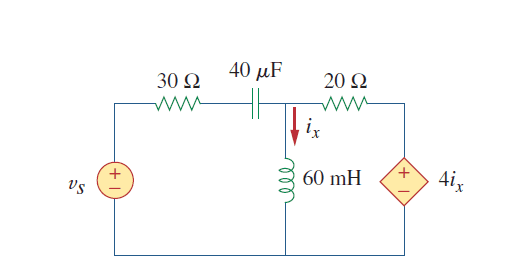# How to do Mesh Analysis with current controlled voltage source

## Homework Statement

Vs = 100cos(2000t)KVL, KCL

## The Attempt at a Solution

My mesh equations are:
-Vs + I1(30-12.5j)-I2(120j)=0
4Ix+I2(120j+20)-I1(120j)=0
Ix = (I1-I2)

Are these correct? When I solve I get a different result than when I do nodal analysis.

gneill
Mentor
Your first mesh equation is missing a term for the potential drop over the inductor due to mesh current ##I1##.

Your first mesh equation is missing a term for the potential drop over the inductor due to mesh current ##I1##.
-Vs + I1(30-12.5j+120j)-I2(120j)=0

i1 = (1.93736+0.385512i)
i2 = (1.83193+0.694348i)

ix = (1.93736+0.385512i)-(1.83193+0.694348i)
V(120j) = ix*120j = 37.06+j12.65 V
The solution (using nodal analysis) says V(120j) = 2.95+j1.126